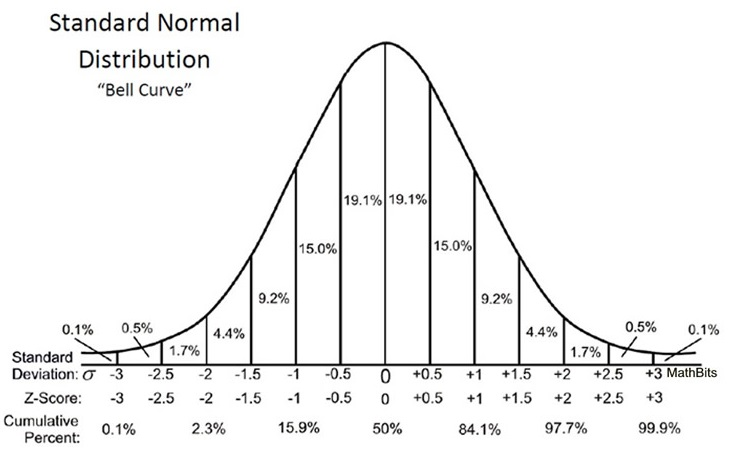# Reply To: What is standard deviation?

Forums General Discussion What is standard deviation? Reply To: What is standard deviation?

“parallax”oldspook –
I’m glad you responded & you are right:).  Standard deviation is a good predictive calculation that is intended to represent the total population of a normal distribution.  Scotchmo’s string #2 is not a normal distribution – I suspect he knows this.  However, it is a very clever & extreme example where standard deviation provided what appears to be better information versus ES.

As always, I need to be more clear in my writing.  I prefer ES for a gun that I have verified has a normal distribution.  Flyers will happen and influence ES – your point is accurate & conceded.

Now I’m no expert on the varying shot string characteristics of the PCP airgun world, but I do know (as most member do) MV is important for accuracy – especially as target distance increases.  So, if one has a gun that shots a normal distribution & longer range accuracy is important, what statistical measure would you put more priority on?

Your answer may vary with regulated versus un-regulated airguns, but please think about it in terms of user refill pressure. What I mean is that most guys refill a PCP at some fixed air tube pressure.  Looking forward to your response.

Clearly you know your maths.  Well written.  And yes scotchmo did give a well contrived (and for sure nearly impossible) counter example.

Whether regulated or unregulated, springer, or multi-pump, if a gun is shooting a normal distribution then the SD is the predictive value you want to place weight upon.  This is because the extreme shot is not predictable.  It could occur now or at the end of the string.  With SD you at least know that 2/3 of your shots will fall withing one SD of the average velocity.  Additionally if that is not sufficiently accurate and you require more precision to hunt humanely, then you limit your range based upon some fraction of the SD which will give you the probability of a hit that you require.  So for example if the SD told me that 2/3 of my shots would fall within 12 fps of the average and that wasn’t good enough I could use the acceptable probability of a hit, say 95%, to estimate the ES that I would have to accept.  That ES would be the ES which could be calculated from the chart here:From that we can see that the ES we would have to accept would be +/- 2 standard deviations from the mean (we wanted 95%).  In our case we would be limited to shooting at our target from ranges where an ES of less than 48 fps would hit the target (our example was SD=12 fps).  Plus or minus 2 standard deviations is plus or minus (2*12) for our example.

Some folks would say that only a 100% probability of a hit is acceptable and so we should consider the ES to be the determining factor.  The ES is a property of the string it represents and is statistically less significant than the SD because it is composed of only 2 samples in the sample set.  If you use the ES of one string, the next string you shoot will almost certainly have a different ES, it might be larger or smaller but because it is only 2 samples from the sample set it will vary more widely than the Standard Deviation from set to set.  So you would probably need to shoot a large number of strings to find out what your true maximum ES was… and then you would be into doing the Standard Deviation of your Extreme Spread… probably more pain than gain at that point.

I want to add this; there is a possibility that the ES of one string falls outside the ES of another string and you can actually compute that probability using the Standard Deviation as the basis of the calculation.  In our example (SD = 12);  The probability of a shot being 36 feet faster than the average is 0.1% (1 in 1000).  The probability of a shot being 36 feet slower than the average is 0.1%(1 in 1000).  The probability of an ES of 72 fps is 0.01%(1 in 10000).  All of this can be read directly from that graph, so nobody has to do that math again.